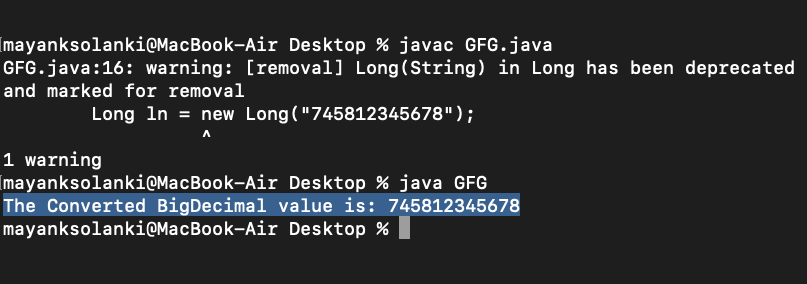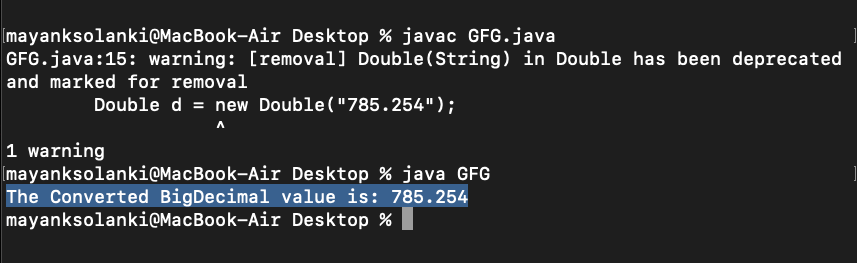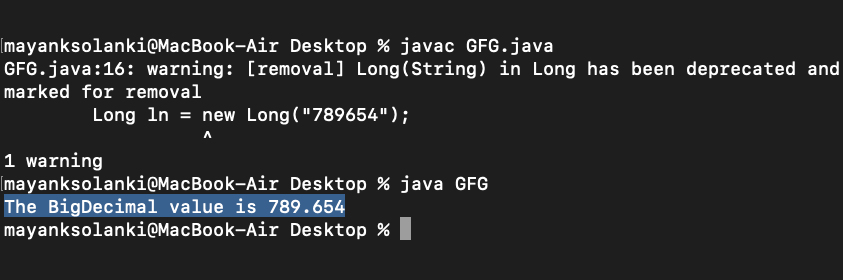# BigDecimal valueOf() Method in Java

• Last Updated : 14 Apr, 2022

java.math.BigDecimal.valueOf(long val) is an inbuilt method in Java that translates a long value into a BigDecimal value with a scale of zero. It allows us, the reuse of frequently used BigDecimal values and hence this “static factory method” is provided in preference to a (long) constructor. Package view is as follows:

```--> java.math Package
--> BigDecimal Class
--> valueOf() Method```

There are 3 types of variations in valueOf() method of BigDecimal class that are as depicted in syntax below via differing in parameters that are as follows:

• public static BigDecimal valueOf(long val)
• public static BigDecimal valueOf(double d)
• public static BigDecimal value(long l, int x)

### Type 1: java.math.BigDecimal.valueOf(long val)

Parameters: The method accepts a single parameter val of Long data type and refers to the value that needs to be translated into a BigDecimal value.

Return value: The method returns a BigDecimal value of Long val.

Example:

## Java

 `// Java Program to Illustrate valueOf() Method``// of BigDecimal Class``// Over long As Arguments`` ` `// Importing required classes``import` `java.math.*;`` ` `// Class``public` `class` `GFG {`` ` `    ``// Main driver  method``    ``public` `static` `void` `main(String[] args)``    ``{`` ` `        ``// Creating and declaring long value by``        ``// creating object of Long class``        ``Long ln = ``new` `Long(``"745812345678"``);`` ` `        ``// Assigning above long value to BigDecimal``        ``BigDecimal b = BigDecimal.valueOf(ln);`` ` `        ``// Printing the BigDecimal value on console``        ``System.out.println(``            ``"The Converted BigDecimal value is: "` `+ b);``    ``}``}`

Output:### Type 2: java.math.BigDecimal.valueOf(double val)

The java.math.BigDecimal.valueOf(double val) is an inbuilt method in java that translates a double into a BigDecimal, using the double’s canonical string representation provided by the Double.toString(double) method.

Syntax:

`public static BigDecimal valueOf(double val)`

Parameters: The method accepts a single parameter val of Double data type and refers to the value that needs to be translated into a BigDecimal value.

Return value: The method returns a BigDecimal value which is equal to or approximately equal to Double val

Example:

## Java

 `// Java Program to Illustrate valueOf() Method``// of BigDecimal Class``// Over Double type As Arguments`` ` `// Importing required classes``import` `java.math.*;`` ` `// Class``public` `class` `GFG {`` ` `    ``// Main driver method``    ``public` `static` `void` `main(String[] args)``    ``{`` ` `        ``// Creating a Double Object``        ``Double d = ``new` `Double(``"785.254"``);`` ` `        ``/// Assigning the bigdecimal value of d to b``        ``BigDecimal b = BigDecimal.valueOf(d);`` ` `        ``// Printing BigDecimal value on console``        ``System.out.println(``            ``"The Converted BigDecimal value is: "` `+ b);``    ``}``}`

Output:### Type 3: java.math.BigDecimal.valueOf(long unscaledVal, int scale)

The java.math.BigDecimal.valueOf(long unscaledVal, int scale) is an inbuilt method in Java that is used to translate a long unscaled value and an int scale into a BigDecimal. This “static factory method” is provided in preference to a (long, int) constructor because it allows for reuse of frequently used BigDecimal values.

Syntax:

`public static BigDecimal valueOf(long unscaledVal, int scale)`

Parameters: It takes two parameters:

• unscaledVal: This is of Long data type and refers to the Unscaled value of the BigDecimal.
• scale: This is of Integer data type and refers to the Scale of the BigDecimal.

Return Value: A big decimal whose value is unscaled*10-scale.

Example:

## Java

 `// Java Program to Illustrate valueOf() Method``// of BigDecimal Class``// Over (long, int) As Arguments`` ` `// Importing required classes``import` `java.math.*;`` ` `// Class``public` `class` `GFG {`` ` `    ``// Main driver method``    ``public` `static` `void` `main(String[] args) {``       ` `        ``// Creating a Long Object by``        ``// creating object of Long class type``        ``Long ln = ``new` `Long(``"789654"``);`` ` `        ``// Assigning the bigdecimal value to``        ``// BigDecimal with scale 3``        ``BigDecimal b = BigDecimal.valueOf(ln, ``3``);`` ` `        ``// Printing BigDecimal value on console``        ``System.out.println(``"The BigDecimal value is "` `+ b);``    ``}``}`

Output:My Personal Notes arrow_drop_up